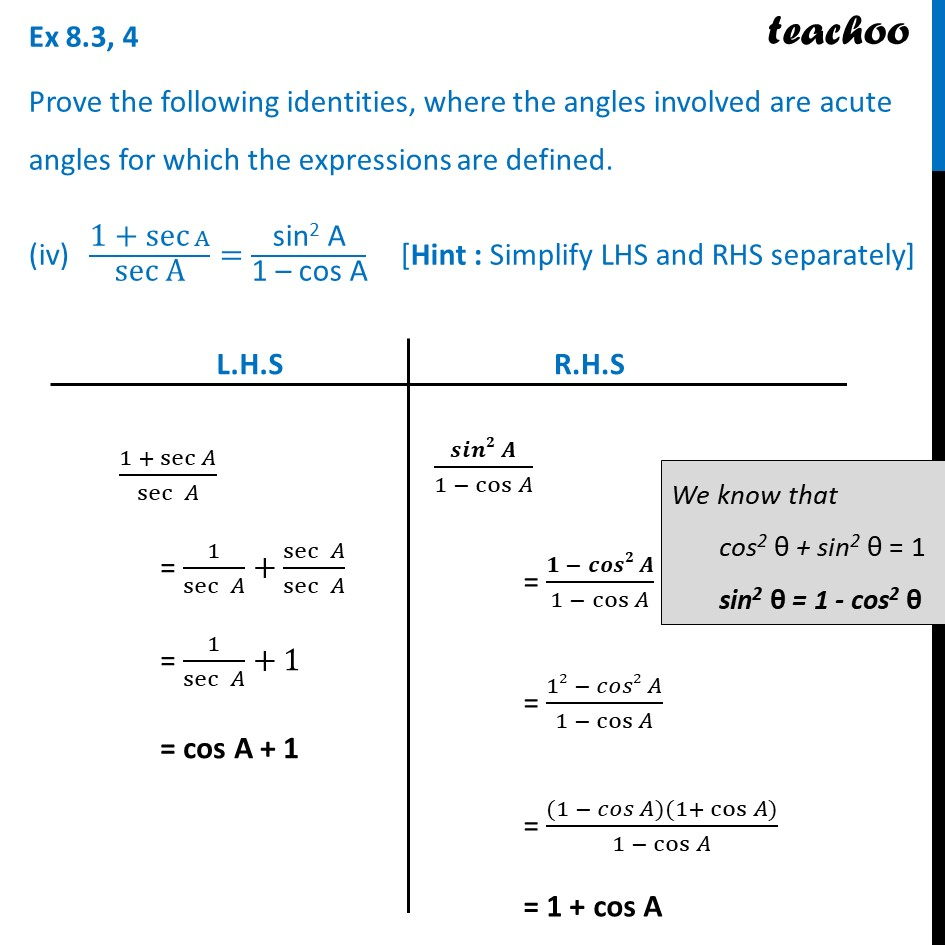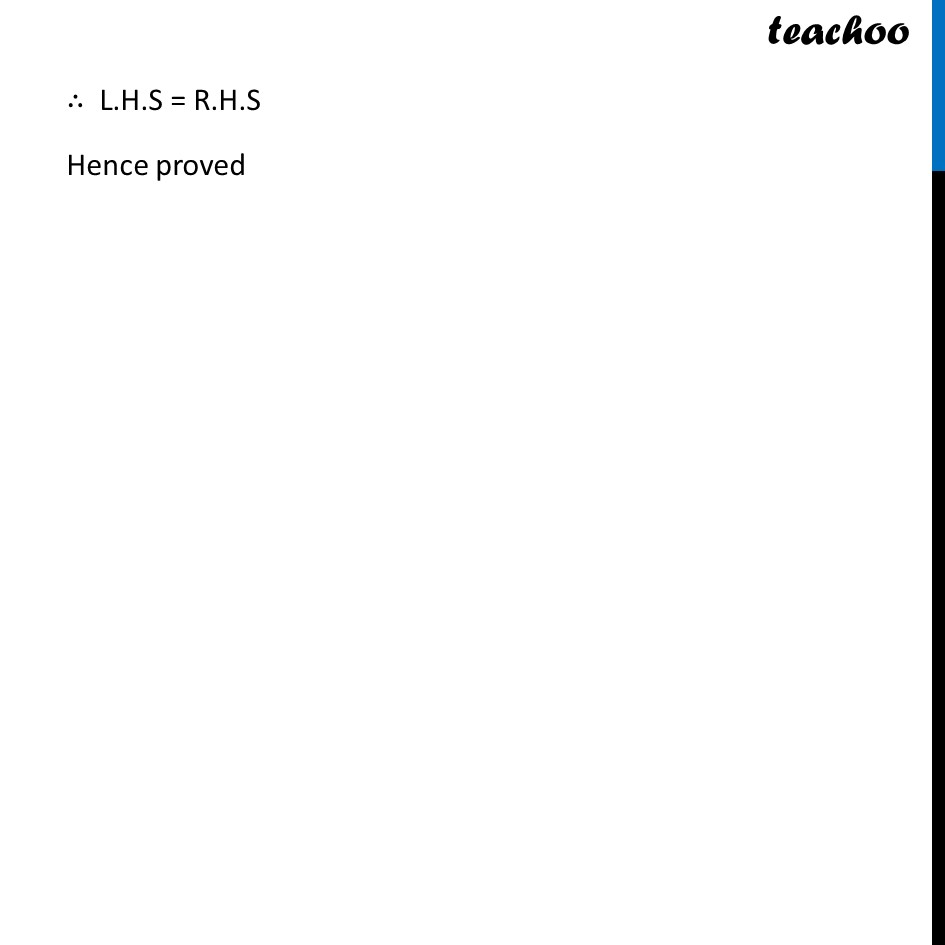Ex 8.3

Chapter 8 Class 10 Introduction to Trignometry
Serial order wiseLearn in your speed, with individual attention - Teachoo Maths 1-on-1 Class

### Transcript

Ex 8.3, 4 Prove the following identities, where the angles involved are acute angles for which the expressions are defined. ("1 + sec" A)/"sec A" ="sin2 A" /"1 – cos A" "[Hint : Simplify LHS and RHS separately]" (1 + sec⁡𝐴)/sec⁡〖 𝐴〗 = 1/sec⁡〖 𝐴〗 +sec⁡〖 𝐴〗/sec⁡〖 𝐴〗 = 1/sec⁡〖 𝐴〗 +1 = cos A + 1 (𝒔𝒊𝒏𝟐 𝑨)/(1 − cos⁡𝐴 ) = (𝟏 − 𝒄𝒐𝒔𝟐 𝑨)/(1 − cos⁡𝐴 ) = (12 − 𝑐𝑜𝑠2 𝐴)/(1 − cos⁡𝐴 ) = ((1 − 𝑐𝑜𝑠 𝐴)(1+ cos⁡〖𝐴)〗)/(1 − cos⁡𝐴 ) = 1 + cos A ∴ L.H.S = R.H.S Hence proved